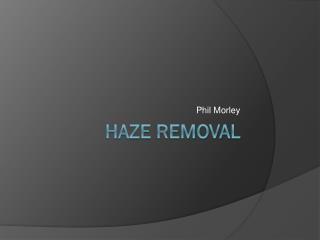DownloadDownload PresentationHaze Removal

# Haze Removal

Download Presentation## Haze Removal

- - - - - - - - - - - - - - - - - - - - - - - - - - - E N D - - - - - - - - - - - - - - - - - - - - - - - - - - -
##### Presentation Transcript

1. Phil Morley Haze Removal

2. The Problem • Fog, Haze, or Smog • Want a clear image • Weather could be common in areas

3. The Method Outlined in paper: Single Haze Removal Using Dark Channel Prior by Kaimin He, Jian Sun, and Xiaoou Tang

4. What is haze? I(x) = J(x)t(x) + A(1 − t(x)) I(x): Image J(x): Scene Radiance A: Atmospheric Light t(x): Transmittance

5. Dark Channel Prior • Objects of interest have low values in at least one color channel • Green leaf • Car Shadow • Dark building • Haze has a high pixel intensity

6. Compute Atmospheric Light • High values in Dark Channel • Take top 0.1% • Pull Values from original image • Average I(x) = J(x)t(x) + A(1 − t(x))

7. Estimating Transmission Shuffling the Haze Equation and taking min’s gives you: Which is simply:

8. Refine Transmission with Soft Matting • Estimated Transmission is blocky • Want to take into account fine detail • Haze Equation is alpha matting • Therefore can use Soft Matting as shown by Levin et al.

9. Soft Matting Minimize Cost Function: Has Closed Form Solution: U3 = 3x3 Identity λ = 0.0001

10. Things to improve • Performance • Processing Time • Memory Allocation • Settings

11. Things To Expand • Depth Map • From Transmittance • 3D Model • Image Enhancement • Histogram Equalization

12. Current Results